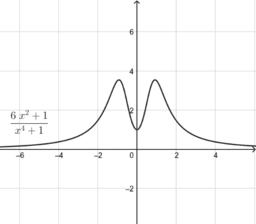# Rational Function Examples

For each graph, describe the end-behavior (horizontal asymptotes if present, or use long division to find the equation of any slant or non-linear asymptotes), the zeros and x-intercepts, the y-intercept, the vertical asymptotes, and use the multiplicity of the factors to decide whether the function changes sign at each x-intercept and vertical asymptote.
•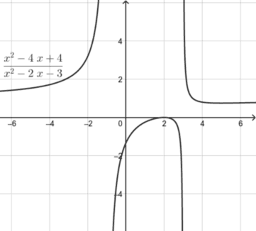### 10.22 Graph A

•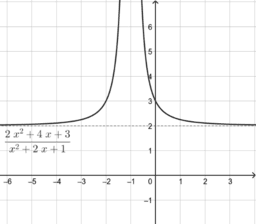### 10.22 Graph B

•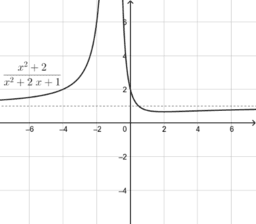### 10.22 Graph C

•### 10.22 Graph D

•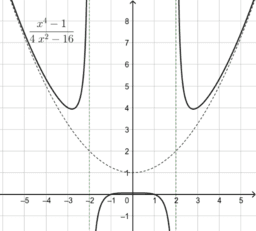### 10.22 Graph E

•### 10.22 Graph F

•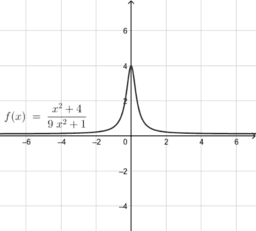### 10.22 Graph G

•